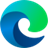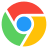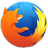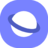# rotateZ() CSS Function

### Description

The CSS `rotateZ()` function is used to rotate an element around the z-axis. This is the axis that goes perpendicular to the screen, from front to back. The function takes a single parameter, which is the angle of rotation in degrees. If the angle is positive, the element will rotate clockwise. If the angle is negative, the element will rotate counter-clockwise.

The `rotateZ()` function can be used to create a variety of effects, such as rotating a 3D object, or rotating a 2D element to give it a 3D appearance. It can also be used to create animations, such as rotating a loading spinner.

Here is an example of how to use the `rotateZ()` function:

``````.element {
transform: rotateZ(45deg);
}
``````

This will rotate the `.element` class 45 degrees clockwise.

The `rotateZ()` function can be used in combination with other CSS transform functions, such as `translateX()`, `translateY()`, and `scale()`, to create more complex effects.

Here is an example of how to use the `rotateZ()` function in combination with the `scale()` function to create a 3D cube:

``````.cube {
transform: rotateZ(45deg) scale(0.5);
}
``````

This will rotate the `.cube` class 45 degrees clockwise and scale it down by half size. This will create the appearance of a 3D cube.

### Syntax

`transform: rotateZ(<angle>);`

### Values

• <angle>A positive value rotates the element clockwise, a negative value rotates the element counter clockwise.

### Example

``````<div class="normal rotated">Rotated</div>
<div class="normal">Not rotated</div>``````
``````.normal {
margin: 20px;
width: 120px;
background: #546D62;
color: white;
font-family: sans-serif;
}
.rotated {
transform: rotateZ(45deg);
}``````

### Browser Support

The following table will show you the current browser support for the CSS `rotateZ()` function.

 Desktop12 12 10 15 4
 Tablets / Mobile18 10 14 3.2 1 3

Last updated by CSSPortal on: 7th October 2023